IF IT'S EDUCATION, IT'S ITS
Pathways To Learning... Since 2005
Hong Kong Registered School 566985

# H - H0, H1 to hypotrochoid - Mathematics Dictionary

A   B   C   D   E   F   G   H   I   J   K   L   M   N   O   P   Q   R   S   T   U   V   W   X   Y   Z

H0 The null hypothesis of a hypothesis test

H1 The alternative hypothesis of a hypothesis test

Hadamard matrix: A square matrix whose entries are either 1 or -1, where the rows (and columns), considered as vectors, are mutually orthogonal..

An example of a 2 x 2 Hadamard matrix is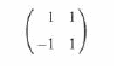half-angle formulae: A set of formulae derived from the double angle formulae, so that trigonometric ratios can be used to find trigonometric ratios with half the angle.

half life The interval of time (or other independent variable) in which the quantity (a dependent variable) in exponential decay is halved.

half-line A (straight) line extending from a point indefinitely in a direction. The equation theta=k for any real values of k in a polar coordinate system describes such a line.

half-plane A (flat) plane extending from a line in a direction.

half-side formulae: Formulae relating the sides and angles of a spherical triangles in spherical trigonometry:where a, b and c are the sides and α, β, and γ are the angles of the spherical triangle, with the usual convention where side a being opposite α, side b opposite β, and side c opposite γ;

where R is found by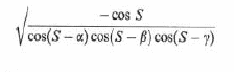and S is half of the sum of the three angles.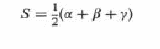half-space One of the two sections that a plane in three dimensions divide into.

halting problem: A important decision problem regarding the determination and determinability of whether a program continues running indefinitely or eventually halts, given an input.

Hamiltonian graph A graph which admits a cycle which visits every vertex of the graph exactly once.

Hamiltonian mechanics A formulation of classical mechanics that is equivalent to Lagrangian mechanics as well as Newtonian mechanics.

Hankel matrix: Informally, a square matrix where each element is always the same as the elements on its top right and bottom left.So that for a matrix M, the element mi,j equals to the elements mi+1,j-1 abd mi-1,j+1 (if they exist).

harmonic analysis: The study of functions and curves which can be represented as the sum (superposition) of waves and related ideas. e.g. Fourier transforms and series

harmonic mean: The harmonic mean of a set of numbers is the reciprocal of the arithmetic mean of the reciprocals of the set of numbers..

harmonic motion: A system with a (negative feedback) force proportional to but in the opposite direction of displacement.

harmonic range: Also known as a harmonic ratio. The symmetric relation between 2 pairs of points where the ratio of the distance of either point in a pair, to both the points in the other pair, is the same.

harmonic sequence: The harmonic sequence is the sequence whose terms are the reciprocals of the positive integers, whereas a harmonic sequence is a sequence whose terms are the reciprocals of the terms of an arithmetic progression.

harmonic series: The series of reciprocals of the positive integers added in order:HCF The highest common factor(between 2 positive integers).

heat equation: A partial differential equation that describes the change in the distribution of heat in a specified region over time.

hectare: An metric unit of area of 10 000 m2.

Hecto-: An SI prefix that means 100.

height The shortest distance between the base (or the infinite extension of the base) of a geometric figure and its vertex or another base (or infinite extension of a base). The height of an obtuse triangle with one of the sides adjacent to the obtuse angle considered to be the base must necessarily be extended to find the shortest distance that is correctly interpreted as the height of the triangle.

helix: A smooth curve in 3-dimensions where the tangent makes a constant angle to a line (the axis), while the angle between the projection of the tangent onto a plane perpendicular to the axis and a line on that plane changes at a constant rate.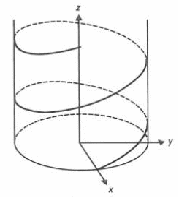hemisphere Either one of the two sections of a sphere divided by a plane through the sphere's centre.

Henry: The SI derived unit of inductance - the ability of an inductor to store energy.

heptagon A polygon with seven sides.

heptahedron(plural heptahedra) A polyhedron with seven faces.

Hermitian conjugate: Also known as the Hermitian transpose, the conjugate transpose or the adjoint. It is the transpose of the matrix of complex conjugate entries of a specified (original) matrix.

Hermitian matrix: A matrix which is its own Hermitian conjugate.

Hero's formula: An equation relating the area of a triangle to its sides: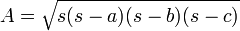with a, b, and c are the lengths of the sides of the triangle and s is half of the perimeter (sum of the lengths of sides)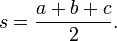Also known as Heron's formula.

Hero's method: An iterative method for refining an approximation of the square root of a number to any accuracy by taking as the new estimate, the arithmetic mean of the previous estimate and the quotient of the target number (the number whose root the method attempts to find) divided by the previous estimate. Also known as Heron's method or the Babylonian method..

hertz The SI unit for the number of cycles per second - frequency. Usually denoted by Hz.

hexadecimal system A base -16 number system. The English letters A, B, C, D, E and F are usually used for the digits 10, 11, 12, 13, 14 and 15 in such a system.

hexagon: A six-sided polygon.

hexahedron: A polyhedron with six faces.

Highest Common Factor: The greatest value in the set of common factor between two numbers.

high quartile: Also known as an upper quartile or third quartile.

hole A type of discontinuity, where the hole is a point whose inclusion into the curve would make the curve continuous.

homogeneous coordinates: MA projective space analogue of the cartesian coordinates for Euclidean geometry. A set of coordinates with the equivalence relation of "being a multiple of" usually with specific rules on representing the equivalence class by one of the members. Thus the number of coordinates is usually one more than the dimension of the projective space.

homogeneous differential equation: 1. A differential equation where all terms are functions (or derivatives) of y.

2. A differential equation where the term not containing derivatives of y is a function whose value remain the same under the (same) scaling of both arguments, i.e. x and y.

homomorphism: A transformation of one set into another that preserves in the second set the relations between elements of the first.

Hopf bifurcation: Also known as a Poincare-Andronov-Hopf bifurcation, it is a type of local bifurcation in a dynamical system.

horizontal: Originally the direction perpendicular to the direction of Earth's gravitation. It can also be simply the direction designated as "horizontal", or perpendicular to directions designated as "vertical" in other situations.

horizontal compression: An enlargement with scale factor between 0 and 1 in the direction designated as horizontal only.

horizontal line equation: The equation representing a constant function - so named due to its representation on a graph.

horizontal line test: A graphical method analogous to the vertical line test, used on graphical representations of mappings to check whether it has an inverse function.

horizontal translation: A translation where the direction is horizontal.

Horner's method: An algorithm for easy evaluation of a polynomial (especially by hand) which relies on the fact that a polynomial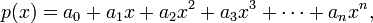of degree n can be thought of as n-1 nested multiplications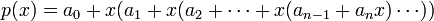This avoids the need to calculate all n powers of the values of x, which may help in reducing the number of steps in calculations (elementary operations), which is of the order n rather than n2.

Hotelling's T-squared distribution: The multi-variate analogue of student's t-distribution.

hour: A unit of time originally defined to be one twenty-fourth (1/24) of a day. As a unit which works with SI units (but not an SI unit itself - base or derived), it is defined as 3600 seconds.

hydrodynamics: Originally, the branch of mathematics now named fluid dynamics. In modern days, it is a sub-branch of fluid dynamics concerned specifically with liquid in motion.

hydrostatics: A sub-branch of fluid mechanics, the mathematical study of liquid in equilibrium.

hyperbola: A curve consisting of the set of points whose distance from a specified point (called the focus) is greater than the distance to a specified line (the directrix) by a fixed proportion. Thus, a hyperbola can be defined by the focus, directrix, and the proportion (called eccentricity - which in the case of hyperbolae must be greater than 1) only. It is one type of conic sections represented by the equation:While the eccentricity can be calculated by:A hyperbola has 2 axis of symmetry, one intersecting the hyperbola (transverse axis) and one not (conjugate axis).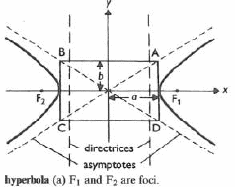hyperbolic functions: Functions related to a hyperbola analogous to how the trigonometric function (also called circular functions) relate to a circle.

These functions are termed hyperbolic sine (sinh), hyperbolic cosine (cosh), hyperbolic tangent (tanh), hyperbolic secant (sech), hyperbolic cosecant (csch), and hyperbolic cotangent (coth).

Hyperbolic sine: sinh x = ½ (ex - e-x)

Hyperbolic cosine: cosh x = ½ (ex + e-x)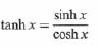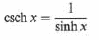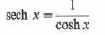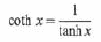Due to the definition, sinh x is an odd function while cosh x is an even function. (Much like their circular counterparts.)

There are also analogous identites: (Related to the circular equivalents by Osborn's Rule)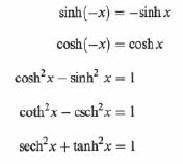The Maclaurin series expansions of sinh x and cosh x are: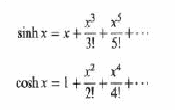And the hyperbolic function relate to the corresponding circular function thus.hyperbolic logarithm: A natural logarithm.

hyperboloid: Surface of revolution by rotating a hyperbola about either one of its two axes of symmetry.

If rotated about the axis which does not intersect the hyperbola, the result is a connected surface.If rotated about the axis which intersects the yperbola (at 2 places), the result is a curve with 2 separate (disconnected) parts.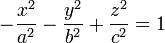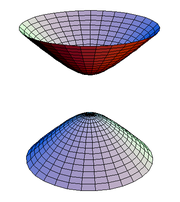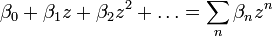where the coefficients βn is an elementary function of the indices.

hypocycloid: The locus of a point on the circumference of a circle rolling inside of another circle. (So that the centre of one is in the other.)

hypotenuse: The side of greatest length in a right-angled triangle, opposite to the right angle.

hypothesis: An unproven (but also not disproven) assertion considered/assumed/proposed to be true.

hypothesis testing: A process of making decision, by considering the probability of a set of observations made given that a certain hypothesis is (or is not) true. The process favours a hypothesis over its inverse and note that the probability used for the judgement of "beyond reasonable doubt" is subjective.

hypotrochoid: A hypotrochoid is the analogous curve to the epitrochoid (much as a hypocycloid is to an epicycloid) where the locus producing point can be anywhere within the inner circle, not necessarily having to be on the circumference of the circle.

A   B   C   D   E   F   G   H   I   J   K   L   M   N   O   P   Q   R   S   T   U   V   W   X   Y   Z

Genius is one percent inspiration and ninety-nine percent perspiration.